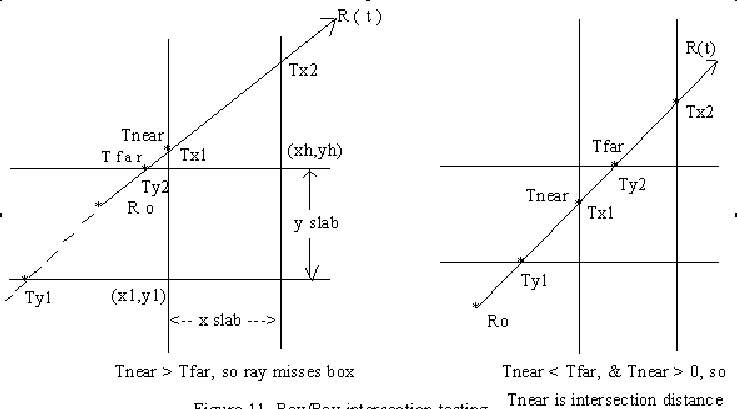# Ray - Box Intersection

We can use a box both as an object and a bounding volume. Kay and Kayjia developed a method based on "slabs" where a slab is the space between two parallel planes. So the intersection of a set of slabs defines a bounding volume or a box.

The method looks at the intersection of each pair of slabs by the ray. It finds tfar and tnear for each pair of slabs. If the overall largest tnear value i.e,. intersection with the near slab, is greater than the smallest tfar value (intersection with far slab) then the ray misses the box, else it hits the box. We can use as the simplest bounding volume the intersection of the sets of two parallel planes where each set has normals along the X, Y, or Z axes.

We will define the box by two coordinates: the box's minimum extent Bl = [ Xl Yl Zl ] and the box's maximum extent Bh = [ Xh Yh Zh ]Image Reference

The Ray is defined as before in terms of Ro, Rd Then the algorithm is as follows:

set Tnear = - infinity, Tfar = infinity

For each pair of planes P associated with X, Y, and Z do:
(example using X planes)
if direction Xd = 0 then the ray is parallel to the X planes, so
if origin Xo is not between the slabs ( Xo < Xl or Xo > Xh) then return false
else, if the ray is not parallel to the plane then
begin
compute the intersection distance of the planes
T1 = (Xl - Xo) / Xd
T2 = (Xh - Xo) / Xd
If T1 > T2 swap (T1, T2) /* since T1 intersection with near plane */
If T1 > Tnear set Tnear =T1 /* want largest Tnear */
If T2 < Tfar set Tfar="T2" /* want smallest Tfar */
If Tnear > Tfar box is missed so return false
If Tfar < 0 box is behind ray return false end

end of for loop If Box survived all above tests, return true with intersection point Tnear and exit point Tfar.

Example: Figure (left)

```
set  tnear =  -infinity,   tfar = infinity
For  X planes
Ray not  parallel
Compute intersection T1 <- Tx1, T2 <- Tx2  ```
```T2 > T1 so no swap
T1 > Tnear so Tnear <-- T1 (Tx1)
T2 < T far  so Tfar <-- Tx2
Tnear < Tfar and  Tfar > 0   so do Y planes

Y planes
Ray not  parallel
Compute intersection  T1 <-- Ty1,  T2 <-- Ty2
T1 < Ty2  so  no  swap
T1  not > Tnear so no change
T2 < Tfar, so Tfar = T2(Ty2)
Tnear > Tfar -> therefore return false and done

Second example from figure (right)

set tnear =  -infinity,   tfar = infinity

X planes
not parallel
t1 <- tx1, t2 <- tx2
If t1 < t2 so no swap;
t1 > tnear so tnear <- t1 ;
t2 < tfar so tfar <- t2;
If tnear < tfar   so OK;
If tfar <  0   so OK;

now do Y planes
not parallel

t1 <- ty1,  t2 <- ty2

t1 < t2 so no swap;
t1 < tnear so no change;
t2 < tfar  so tfar <- t2;
tnear > tfar  ? no
tfar < 0      ?  no

Thus, return true with intersection point = tnear = tx1;```

Last changed April 01, 1998, G. Scott Owen, owen@siggraph.org